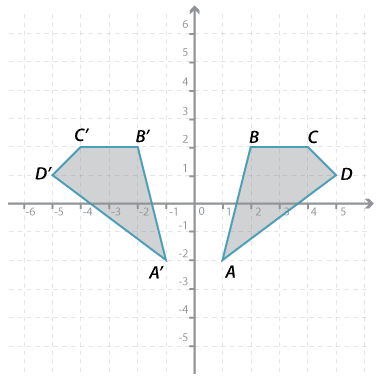#### Question 3Detailed description

The quadrilateral $$ABCD$$ is reflected in the $$y$$-axis to form rectangle $$A^\prime B^\prime C^\prime D^\prime$$.

Give the $$x$$-coordinate and the $$y$$-coordinate of each of the following:

$$x$$ = $$y$$ = Submit answer
a $$A^\prime$$ ( , ).
b $$B^\prime$$ ( , ).
c $$C^\prime$$ ( , ).
d $$D^\prime$$ ( , ).

Place answers in both checkboxes before submitting.

#### Question 4

The rectangle $$ABCD$$ is reflected in the $$y$$-axis to form rectangle $$A^\prime B^\prime C^\prime D^\prime$$. The coordinates of each of the vertices of $$ABCD$$ are as follows:

$$A(1, 6), B(1, 9), C(2, 9), D(2, 6)$$.

Give the $$x$$-coordinate and the $$y$$-coordinate of:

$$x$$ = $$y$$ = Submit answer
a $$A^\prime$$ ( , ).
b $$B^\prime$$ ( , ).
c $$C^\prime$$ ( , ).
d $$D^\prime$$ ( , ).

Place answers in both checkboxes before submitting.